# Methods and formulas for Moving Average Chart

Select the method or formula of your choice.

## Plotted points

when i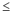v
when i > v

### Notation

TermDescription
vnumber of sample means that are averaged
μprocess mean
kparameter for Test 1. The default is 3.
σprocess standard deviation
nisize of subgroup i

## Center line

The center line represents the process average. Minitab uses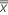to estimate μ.

## Control limits

### Lower control limit (LCL)

when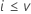when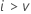### Upper control limit (UCL)

whenwhen### Notation

TermDescription
nisize of subgroup i
vnumber of sample means that are averaged
μprocess mean
kparameter for Test 1. The default is 3.
σprocess standard deviation

## Methods and formulas for Box-Cox

### Box-Cox formula

If you use a Box-Cox transformation, Minitab transforms the original data values (Yi) according to the following formula:

where λ is the parameter for the transformation. Minitab then creates a control chart of the transformed data values (Wi). To learn how Minitab chooses the optimal value for λ, go to Methods and formulas for Box-Cox Transformation.

### Common λ values

The following table shows some commonly used λ values and their transformations.
λ Transformation
2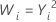0.5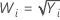0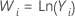−0.5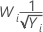−1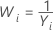By using this site you agree to the use of cookies for analytics and personalized content.  Read our policy